## Li's Research Page

Last updated: May 2000

My primary research interests are in computational geometry and its interaction with the subjects that deal with the physical world, such as computer graphics, dynamic simulation, robotics, and computational molecular biology. In general, my goal is to

• understand the geometric computing in computational tasks involving physical world, and
• provide algorithmic and analysis tools for handling geometric objects.

Moving or deforming objects are common in physical processes; and the traditional algorithmic paradigms are challenged by the modeling of geometric shapes and relationships of such objects. In the past years, my research has mainly focused on designing algorithms for moving/deforming objects under the kinetic data structure framework, a novel algorithmic paradigm proposed by Basch, Guibas, and Hershberger in 1997. Under the kinetic data structure framework, a geometric structure is maintained in an event driven manner where the structure is certified by a set of primitive conditions and examined only at the times when one of the conditions fails; or in other words, a geometric structure is maintained by animating a proof of correctness over time.

In my work, I show how to design efficient kinetic data structures for a large collection of geometric problems. Specifically, the structures studied are the proximity, separation, and visibility structures that arise naturally from the application areas to answer the fundamental questions such as which objects are close to a given object, whether an object collides with other objects, and whether an object is occluded by other objects, etc.

I also work on the practical and theoretical issues related to kinetic data structures such as evaluating KDS in practice, applying KDSs to dynamic simulations, analyzing the combinatorial changes of geometric structures under probabilistic models, designing robust KDSs, and exploiting the connection between kinetic proofs and other algorithmic proofs.

Besides geometric computing, I am also very interested in broad areas of algorithms, cryptography, and computer security. Here is the summary of the work that I have done in these areas.

Proximity Structures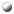Proximity Problems on Moving Points. with J. Basch and L. Guibas. Proceeding of 13th ACM Symposium on Computational Geometry, 1997.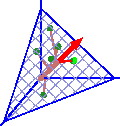Abstract. A kinetic data structure for the maintenance of a multidimensional range search tree is introduced. This structure is used as a building block to obtain kinetic data structures for two classical geometric proximity problems in arbitrary dimensions: the first structure maintains the closest pair of a set of continuously moving points, and is provably efficient. The second structure maintains a spanning tree of the moving points whose cost remains within some prescribed factor of the minimum spanning tree. Download [ conference version | full version ]Euclidean Proximity and Power Diagrams. with L. Guibas. Proceeding of 10th Canadian Conference on Computational Geometry, 1998.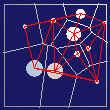Abstract. Power diagrams are a useful generalization of Voronoi diagrams in which the sites defining the diagram are not points but balls. Most recently power diagrams have played a key role in work related to alpha shapes and `skins', as collections of balls are a natural model for molecular structures. The Voronoi diagram of point sites in any dimension has many useful proximity properties. In this paper, we consider the the power diagram of a set of disjoint balls. We show that the closest pair of balls (in Euclidean sense) are neighbors in the power diagram, while the nearest neighbor to a given ball is not necessarily a power diagram neighbor. This property gives us a way to maintain the closest pair among a set of moving balls by maintaining (dual of) the power diagram. As long as the moving balls are disjoint, maintaining the power diagram is similar to maintaining the Voronoi diagram of moving points. In both cases local certificates prove the correctness of the structure; when one of them fails, an easy update restores the correctness of the structure. DownloadCompact Voronoi Diagrams for Moving Convex Polygons. with L. Guibas and J. Snoeyink. To appear in 7th Scandinavian Worshop on Algorithm Theory, 2000.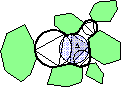Abstract. We describe a kinetic data structure for maintaining a compact Voronoi-like diagram of convex polygons moving around in the plane. We use a compact diagram for the polygons, dual to the Voronoi. A key feature of this diagram is that its size is only proportional to the number of polygons and not to their complexity. We demonstrate a local certifying property of that diagram, akin to that of Delaunay triangulations of points. We then obtain a method for maintaining this diagram that is output-sensitive and costs O(log n) per update. Furthermore, we show that for a set of k polygons with a total of \$n\$ vertices moving along bounded degree algebraic motions, this dual diagram, and thus their compact Voronoi diagram, changes combinatorially Ω(n2) and roughly O(kn2) times. This compact Voronoi diagram can be used for collision detection or retraction motion planning among the moving polygons. DownloadKinetic Connectivity for Unit Disks. with L. Guibas, J. Hershberger, and S. Suri. To appear in 16th ACM Symposium on Computational Geometry, 2000.Abstract. We describe a kinetic data structure (KDS) that maintains the connected components of the union of a set of unit-radius disks moving in the plane. We assume that the motion of each disk can be specified by a low-degree algebraic trajectory; this trajectory, however, can be modified in an on-line fashion. While the disks move continuously, their connectivity changes at discrete times. Our main result is an linear space data structure that takes logarithmic time per connectivity query of the form "are disks A and B in the same connected component?" A straightforward approach based on dynamically maintaining the overlap graph requires Ω(n2) space. Our data structure requires only linear space and deal with roughly quadratically many updates in the worst case, each requiring O(log2 n) amortized time. This number of updates is close to optimal, since a set of n moving unit disks can undergo quadratically many connectivity changes. DownloadHWalk: Hierarchicial method for Real-time Distance Computation Among Moving Convex Bodies. with L. Guibas and D. Hsu. In Proc. 15th ACM Symposium on Computational Geometry, pp. 344-351, 1999. The full version in Computational Geometry: Theory and Applications, Vol. 15(1-3):51-68, 2000.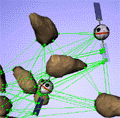Abstract. This paper presents the Hierarchical Walk, or HWalk algorithm, which maintains the distance between two moving convex bodies by exploiting both motion coherence and hierarchical representations. For convex polygons, we prove that HWalk improves on the classic Lin-Canny and Dobkin-Kirkpatrick algorithms. We have implemented HWalk for moving convex polyhedra in three dimensions. Experimental results indicate that, unlike previous incremental distance computation algorithms, HWalk adapts well to variable coherence in the motion and provides consistent performance. Download [ conference version | journal version ] Summary

Separation StructuresKinetic Collision Detection for Two Simple Polygons. with J. Basch, J. Erickson, L. Guibas, and J. Hershberger. Proceeding of 10th Annual ACM-SIAM Symposium on Discrete Algorithms, 1999.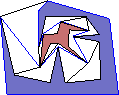Abstract. We design a kinetic data structure for detecting collisions between two simple polygons in motion. In order to do so, we create a planar subdivision of the free space between the two polygons, called the external relative geodesic triangulation, which certifies their disjointness. We show how this subdivision can be maintained as a kinetic data structure when the polygons are moving, and analyze its performance in the kinetic setting. DownloadSeparation-Sensitive Convex Collision Detection. with J. Erickson, L. Guibas, and J. Stolfi. Proceeding of 10th Annual ACM-SIAM Symposium on Discrete Algorithms, 1999.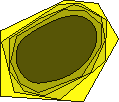Abstract. We develop a class of new kinetic data structures for collision detection between moving convex polytopes; the performance of these structures is sensitive to the separation of the polytopes during their motion. For two convex polygons in the plane, let D be the maximum diameter of the polygons, and let s be the minimum distance between them during their motion. The changes of our separation certificate can be expressed as a function of D/s. Such motion-sensitive analysis is very useful in practice where the worst case analysis is usually insufficient. Variants of these data structures are also shown that exhibit hysteresis---after a separation certificate fails, the new certificate cannot fail again until the objects have moved by some constant fraction of their current separation. We can then bound the number of events by the combinatorial size of a certain cover of the motion path by balls. DownloadDeformable Free Space Tilings for Kinetic Collision Detection. with P. Agarwal, J. Basch, L. Guibas, and J. Hershberger. To appear in 4th International Workshop on Algorithmic Foundations of Robotics, 2000.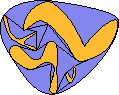Abstract. We present kinetic data structures for detecting collisions between a set of polygons that are not only moving continuously but whose shapes can also vary continuously with time. Unlike classical collision detection methods that rely on bounding volume hierarchies, our method is based on deformable tilings of the free space surrounding the polygons. The basic shape of our tiles is that of a pseudo-triangle, a shape sufficiently flexible to allow extensive deformation, yet structured enough to make detection of self-collisions easy. We show different schemes for maintaining pseudo-triangulations as a kinetic data structure, and we analyze their performance. Specifically, we first describe an algorithm for maintaining a pseudo-triangulation of a point set, and show that the pseudo-triangulation changes only quadratically many times if points move along algebraic arcs of constant degree. We then describe an algorithm for maintaining a pseudo-triangulation of a set of convex polygons. Finally, we extend our algorithm to the general case of maintaining a pseudo-triangulation of a set of moving or deforming simple polygons. Download [ conference version | full version ] Summary

Visibility StructuresVisibility Queries in Simple Polygons and Applications. with B. Aronov, L. Guibas, and M. Teichmann. In Proc. 9th Annual International Symposium on Algorithms and Computation, 1998.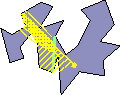Abstract. In this paper we explore some novel aspects of visibility for stationary and moving points inside a simple polygon. We provide a mechanism for expressing the visibility polygon from a point as the disjoint union of logarithmically many canonical pieces using a quadratic-space data structure. This allows us to report visibility polygons in time proportional to their size, but without the cubic space overhead of earlier methods. The same canonical decomposition can be used to determine visibility within a frustum, or to compute various attributes of the visibility polygon efficiently. By exploring the connection between visibility polygons and shortest-path trees, we obtain a kinetic algorithm that can track the visibility polygon as the viewpoint moves along polygonal paths inside the polygon, at a poly-logarithmic cost per combinatorial change in the visibility or in the flight plan of the point. The combination of the static and kinetic algorithms leads to a new static algorithm in which we can trade off space for increased overhead in the query time. As another application, we obtain an algorithm which computes the weak visibility polygon from a query segment in output-sensitive time. DownloadOn Incremental Rendering of Silhouette Maps of a Polyhedral Scene. with A. Efrat, L. Guibas, and O. Hall-Holt. To appear in Proceeding of 11th Annual ACM-SIAM Symposium on Discrete Algorithms, 2000.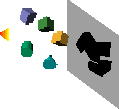Abstract. We consider the problem of incrementally rendering a polyhedral scene while the viewpoint is moving. In practical situations the number of geometric primitives to be rendered can be very large --- hundreds of thousands or millions, but these may come from only a moderate number of objects that happen to have been finely tessellated. It is sometimes advantageous to render only the silhouettes of the objects, rather than the objects themselves, and then exploit coherence or other methods to optimize the rendering of single-object regions with uniform reflectance properties. Such an approach is also regularly used in the domain of non-photorealistic rendering, where the rendering of silhouette edges plays a key role. The hard part in efficiently implementing a kinetic approach to this problem is to realize when the rendered silhouette undergoes a combinatorial change. We assume that our objects are convex polytopes, and that the viewpoint is moving along a straight line, or along an algebraic curve. The resulting bounds will then depend on both the number of objects, and the total number of edges, in such a way that we can describe the advantages of focusing on the silhouettes of combinatorially large objects. We also study the special case when the scene is a polyhedral terrain, and present improved bounds for this case. In addition to event bounds, we also obtain algorithms that compute all the changes occurring during a linear motion, (both for general scenes and for terrains). Download Summary

Other KDS topicsA Practical Evaluation of Kinetic Data Structures. with J. Basch, L. Guibas, and C. Silverstein. Proceeding of 13th ACM Symposium on Computational Geometry, pp. 388-390, 1997.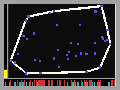Abstract. Much study has been devoted to updating geometric structures as they change over time. Most of this research has concerned efficient insertion and deletion of objects, but a recent paper [BGH-97] has developed kinetic data structures for problems such as the convex hull and closest pair of points moving in the plane. These are data structures that can be easily updated as objects move continuously in space. In that paper, however, only worst-case measures for these structures were derived, and these might not reflect how the kinetic structures really perform in practice. In this paper, we study the data structures of [BGH-97] and compare them against alternative kinetic structures and other simple, brute force methods. We use this data to develop empirical bounds on the performance of the kinetic data structures verifying that on a wide range of problems they are superior to alternative approaches. We also address numerical robustness issues for kinetic data structure implementation. Download [ conference version | full version ]Probabilistic Analysis for Combinatoral Functions of Moving Points with J. Basch, H. Devarajan, and P. Indyk. Proceeding of 13th ACM Symposium on Computational Geometry, pp. 442-444, 1997. Full version to appear in International Journal of Computational Geometry and Applications.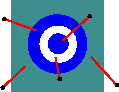Abstract. We initiate a probabilistic study of configuration functions of moving points. In our probabilistic model, a particle is given an initial position and a velocity drawn independently at random from the same distribution D. We show that if n particles are drawn independently at random from the uniform distribution on the square, their convex hull undergoes O(log2n) combinatorial changes in expectation, their Voronoi diagram undergoes O(n3/2) combinatorial changes, and their closest pair undergoes O(n) combinatorial changes. We also consider the probabilistic analysis of the efficiency of kinetic data structures. Download [ conference version | full version ]

Work in Other AreasFault tolerant networks with small degree. To appear in 11th ACM Symposium on Parallel Algorithms and Architectures, 2000. Abstract. In this paper, we study the design of fault tolerant networks for arrays and meshes by adding redundant nodes and edges. For a target graph \$G\$ (linear array or mesh in this paper), a graph \$G'\$ is called a \$k\$-fault-tolerant graph of \$G\$ if when we remove any \$k\$ nodes from \$G'\$, it still contains a subgraph isomorphic to \$G\$. The major quality measures for a fault-tolerant graph are the number of spare nodes it uses and the maximum degree it has. The degree is particularly important in practice as it poses constraints on the scalability of the system. In this paper, we aim at designing fault-tolerant graphs with both small degree and small number of spare nodes. The graphs we obtain have degree \$O(1)\$ for arrays and \$O(\log^3 k)\$ for meshes. The number of spare nodes used are \$O(k\log^2 k)\$ and \$O(k^2/\log k)\$, respectively. Compared to the previous results, the number of spare nodes used in our construction has one fewer linear factor in \$k\$. DownloadOn the Time-Randomness Tradeoff for Oblivious Routing. with Y. Dai and J. Shang. Chinese Journal of Computers, Vol. 19, No. 5, pp. 388-397. 1996. Abstract. In oblivious routing, the route for each packet is determined by its source and destination. In the seminal paper by Valiant, it is shown that randomness can help to significantly reduce the time steps needed to route a permutation for oblivious routing --- in randomized oblivious routing, the route of a packet is picked from a set of paths according to a predetermined probabilistic distribution. In this paper, we show a tradeoff between the random bits needed and the routing latency in distributed oblivious routing. In particular, our result implies that the number of random bits used in Valiant's algorithm is optimal asymptotically.Efficient Systolic Implementation of RSA Operations. with K. Lu. In Proceeding of 3rd Annual Symposium on Computer Information Security, Chinese Computer Association, 1993. Abstract. In this paper, we present efficient systolic implementations for large number arithmetics used in RSA en/decryptions.Secret exchange without computational hardness assumption. with W. Chen and J. He. In Proceeding of 2nd National Conference of Young Scientists, Beijing, China, 1992. Abstract. In this paper, we study the problem of exchanging secrets in public channel without computational hardness assumption. We are able to design schemes to against adversaries with similar computing power,An efficient public key agreement scheme. with B. Jiang. In Proceeding of 2nd Annual Symposium on Computer Information Security, Chinese Computer Association, 1992. Abstract. A framework is proposed for exchange secret based on one-way abel group operations. Under this framework, we design new secret exchange methods based on families of matices that are commutative under multiplication.An identity based dynamic password verification scheme. In Proceeding of 2nd Annual Symposium on Computer Information Security, Chinese Computer Association, 1992. Abstract. As title.

Work in ProgressCollision Detection of Deformable Objects. with B. Mirtich.Distributed Trust System. with J. He.

Publication List

1. Fault-tolerant networks with small degree. To appear in 11th ACM Symposium on Parallel Algorithms and Architectures, 2000.

2. Kinetic data structures for efficient simulation, with L. Guibas and F. Xie. To appear, 2000.

3. Compact Voronoi diagram for moving convex polygons, with L. Guibas and J. Snoeyink. To appear in 7th Scandinavian Worshop on Algorithm Theory, 2000.

4. Kinetic connectivity for unit disks, with L. Guibas, J. Hershberger, and S. Suri. To appear in 16th ACM Symposium on Computational Geometry, 2000.

5. Deformable free space tiling for kinetic collision detection, with P. Agarwal, L. Guibas, and J. Hershberger. To appear in 4th International Workshop on Algorithmic Foundations of Robotics, 2000. full version

6. On incremental rendering of silhouette maps of a polyhedral scene, with A. Efrat, L. Guibas, and O. Hall-Holt. In Proc. 11th Annual ACM-SIAM Symposium on Discrete Algorithms, 2000.

7. A hierarchical method for real-time distance computation among moving convex bodies, with L. Guibas, and D. Hsu. To appear in Special Issue on Virtual Reality, Computational Geometry: Theory and Applications.

8. H-Walk: hierarchical distance computation for moving convex bodies, with L. Guibas and D. Hsu. In Proc. 15th ACM Symposium on Computational Geometry, pp. 344-351, 1999.

9. Kinetic collision detection for two simple polygons, with J. Basch, J. Erickson, L. Guibas, and J. Hershberger. In Proc. 10th Annual ACM-SIAM Symposium on Discrete Algorithms, pp. 102-111, 1999.

10. Separation-sensitive convex collision detection, with J. Erickson, L. Guibas, and J. Stolfi. In Proc. 10th Annual ACM-SIAM Symposium on Discrete Algorithms, pp. 327-336, 1999. To appear in Computational Geometry: Theory and Applications.

11. Visibility queries in simple polygons and applications, with B. Aronov, L. Guibas, and M. Teichmann. In Proc. 9th Annual International Symposium on Algorithms and Computation, pp. 357-366, 1998.

12. Euclidean proximity and power diagrams, with L. Guibas. In Proc. 10th Canadian Conference on Computational Geometry, 1998.

13. Proximity problems on moving points, with J. Basch and L. Guibas. In Proc. 13th ACM Symposium on Computational Geometry, pp. 344-351, 1997. full version

14. A practical evaluation of kinetic data structures, with J. Basch, L. Guibas, and C. Silverstein. In Proc. 13th ACM Symposium on Computational Geometry, pp. 388-390, 1997. full version

15. Probabilistic analysis for combinatorial functions of moving points, with J. Basch, H. Devarajan, and P. Indyk. In Proc. 13th ACM Symposium on Computational Geometry, 442-444, 1997. Full version to appear in International Journal of Computational Geometry and Applications.

16. On the time-randomness tradeoff for oblivious routing, with Y. Dai and J. Shang. Chinese Journal of Computers, Vol. 19, No. 5, pp. 388-397. 1996.

17. Efficient systolic implementation of RSA operations, with K. Lu. In Proceeding of 3rd Annual Symposium on Computer Information Security, Chinese Computer Association, 1993.

18. Secret exchange without computational hardness assumption, with W. Chen and J. He. In Proceeding of 2nd National Conference of Young Scientists, Beijing, China, 1992.

19. An efficient public key agreement scheme, with B. Jiang. In Proceeding of 2nd Annual Symposium on Computer Information Security, Chinese Computer Association, 1992.

20. An identity based dynamic password verification scheme. In Proceeding of 2nd Annual Symposium on Computer Information Security, Chinese Computer Association, 1992.

Softwares

Co-authors

My Erdos number is two since one of my coauthors wrote a paper with Erdos!